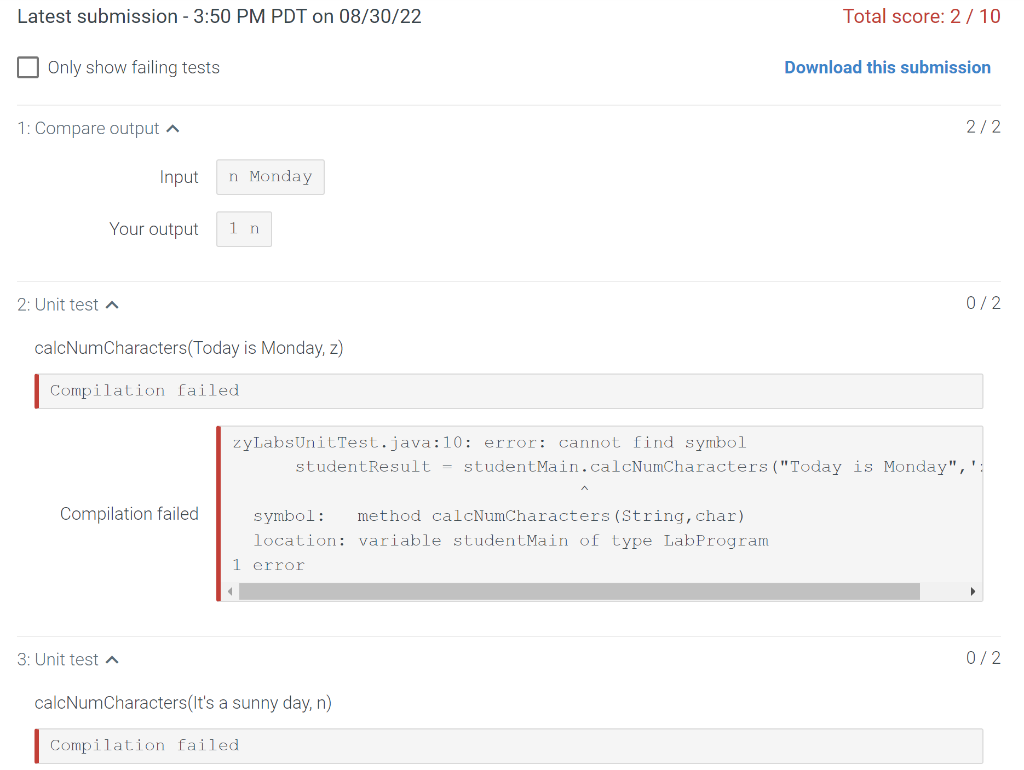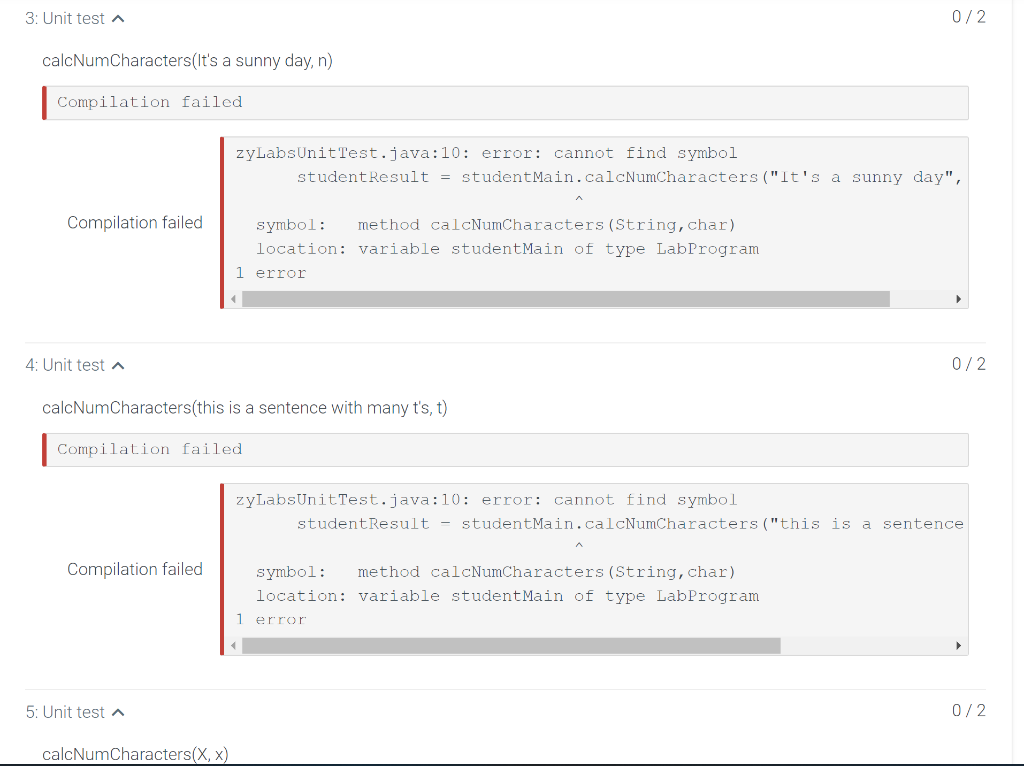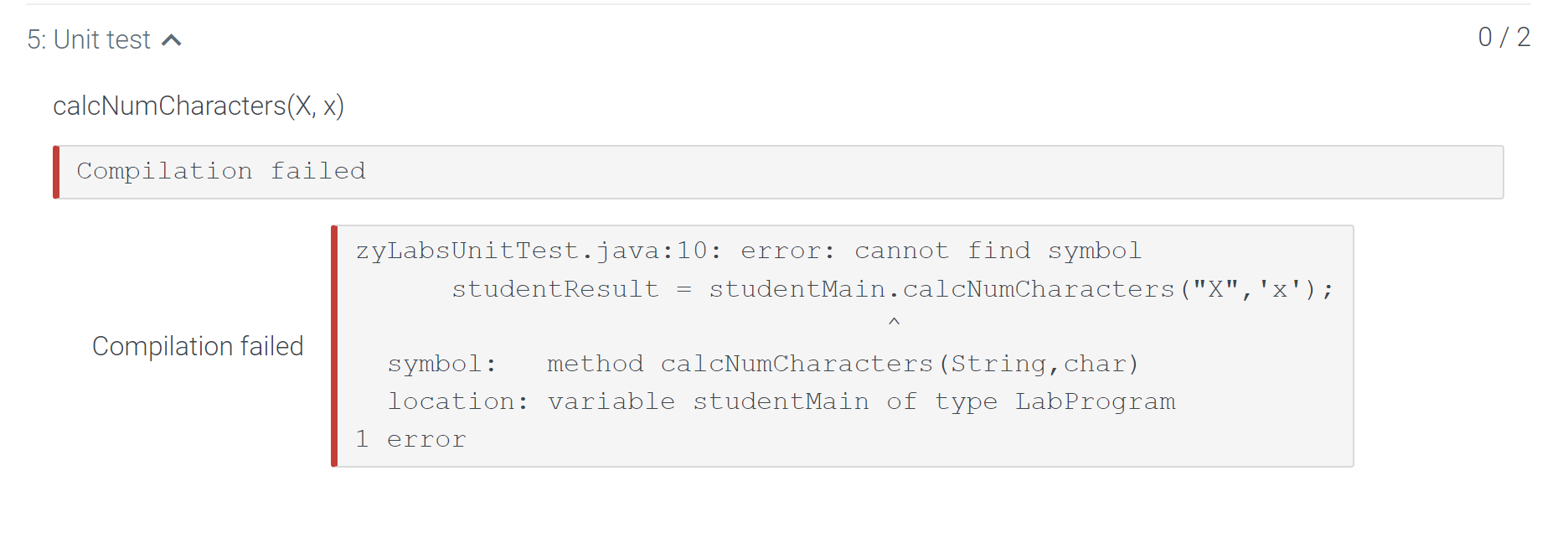# (Solved): 7.8 LAB: Count characters - methods These are the instructions for the code, I have already wor ...

7.8 LAB: Count characters - methods

These are the instructions for the code, I have already worked out a code but I get errors, I provide the errors in the images below, I would like to get those erros fixed, the code that i provide is the one i worked out

Write a program whose input is a character and a string, and whose output indicates the number of times the character appears in the string. The output should include the input character and use the plural form, n's, if the number of times the characters appears is not exactly 1.

Ex: If the input is:

n Monday


the output is:

1 n


Ex: If the input is:

z Today is Monday


the output is:

0 z's


Ex: If the input is:

n It's a sunny day


the output is:

2 n's


Case matters. n is different than N.

Ex: If the input is:

n Nobody


the output is:

0 n's


The program must define and call the following method that takes the input string and character as parameters, and returns the number of times the input character appears in the input string.
public static int calcNumCharacters(String userString, char userChar)

import java.util.Scanner;

public class LabProgram {
public static int countCharacters(char userChar, String userString) {
int count = 0;

for (int i = 0; i < userString.length(); i++) {
if (userString.charAt(i) == userChar) {
count++;
}
}

return count;
}

public static void main(String[] args) {
Scanner scnr = new Scanner(System.in);
char userChar = scnr.next().charAt(0);
String userString = scnr.nextLine();
scnr.close();

int count = countCharacters(userChar, userString);
if(count == 1){
System.out.println(count+" "+userChar);
}
else{
System.out.println(count+" "+userChar+"'s");
}

}

}
}Latest submission - 3:50 PM PDT on $$08 / 30 / 22$$ Total score: $$2 / 1$$ Only show failing tests Download this submission 1: Compare output $$\boldsymbol{\wedge}$$ Input Your output 2: Unit test ? calcNumCharacters(Today is Monday, z) Compilation failed zyLabsUnitTest. java: 10: error: cannot find symbol studentResult - studentMain. calcNumCharacters ("Today is Monday", , symbol: method calcNumCharacters (String, char) location: variable studentMain of type LabProgram 1 error 3: Unit test $$\boldsymbol{\wedge}$$ calcNumCharacters(It's a sunny day, n) 3: Unit test $$\boldsymbol{\wedge}$$ $$0 / 2$$ calcNumCharacters(It's a sunny day, n) Compilation failed \begin{tabular}{r|r} zyLabsunitTest.java:10: error: cannot find symbol \\ studentResult = studentMain. calcNumCharacters ("It's a sunny day", \\ Compilation failed \\ symbol: method calcNumCharacters (String, char) location: variable studentMain of type LabProgram 1 error \end{tabular} 4: Unit test $$\boldsymbol{\wedge}$$ calcNumCharacters(this is a sentence with many t's, $$t$$ ) Compilation failed \begin{tabular}{c|l} zyLabsUnitTest.java: 10: error: cannot find symbo $$\perp$$ \\ studentResult - studentMain. calcNumCharacters ("this is a sentence \end{tabular} zyLabsUnitTest. java:10: error: cannot find symbol studentResult = studentMain. calcNumCharacters ("X", ' $$\left.\mathrm{X}^{\prime}\right)$$; symbol: method calcNumCharacters (string, char) location: variable studentMain of type LabProgram 1 error

We have an Answer from Expert